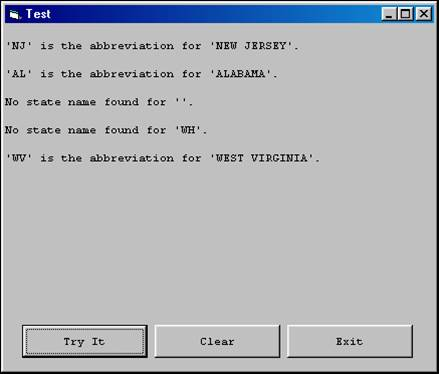Arrays

Searching

When working with arrays, it is often necessary to perform a search or "lookup" to determine whether an array contains a value that matches a certain key value. For example, the data from a file or database table will typically contain a "key" field (such as a code), along with corresponding data fields (such as a description). It is often convenient to work with such data by first loading it into a UDT array in an initial routine, and then later pulling data from the array given a key value to search on.

Two versions of a sample project to look up a state abbreviation and return the corresponding state name will now be presented. The first version demonstrates how to perform a serial search (also called a linear search) on an array; the second demonstrates how to perform a binary search.

These projects will process a sequential file of state abbreviations and corresponding state names called STATES.DAT, with the following contents:

"AL","ALABAMA"

.

.

.

"WV","WEST VIRGINIA"

"WY","WYOMING"

Serial Search Example

Following is the step-by-step process to build this project.

·         Start a new "Try It" project.

• In the general declarations section, place the following code:

Option Explicit

Private Type StateRecord

StateAbbrev  As String

StateName    As String

End Type

Dim maudtStateRecord()  As StateRecord

• Place the following code in the Form_Load event to initialize the state record array with the data from the file:

Dim intStateFileNbr As Integer

Dim intStateUB      As Integer

Dim strBS           As String

strBS = IIf(Right\$(App.Path, 1) = "\", "", "\")

intStateFileNbr = FreeFile

intStateUB = -1

Open (App.Path & strBS & "STATES.DAT") For Input As #intStateFileNbr

Do Until EOF(intStateFileNbr)

intStateUB = intStateUB + 1

ReDim Preserve maudtStateRecord(0 To intStateUB)

With maudtStateRecord(intStateUB)

Input #intStateFileNbr, .StateAbbrev, .StateName

End With

Loop

End Sub

• Place the following code int cmdTryIt_Click event:

Private Sub cmdTryIt_Click()

Dim strTestAbbrev  As String

Dim strTestName    As String

strTestAbbrev = UCase\$(InputBox("Enter a state abbreviation.", _

"State Lookup Test"))

strTestName = GetStateName(strTestAbbrev)

Print

If strTestName = "" Then

Print "No state name found for '" & strTestAbbrev & "'."

Else

Print "'" & strTestAbbrev & "' is the abbreviation for '" _

& strTestName & "'."

End If

End Sub

·         Code the GetStateName function, which uses a serial search to find the state name, given the state abbreviation:

Private Function GetStateName(pstrTestAbbrev As String) As String

Dim intX  As Integer

For intX = 0 To UBound(maudtStateRecord)

If pstrTestAbbrev = maudtStateRecord(intX).StateAbbrev Then

GetStateName = maudtStateRecord(intX).StateName

Exit Function

End If

Next

GetStateName = ""

End Function

The technique used here is called a serial search, because the StateAbbrev elements of the maudtStateRecord UDT array are compared one by one to the state abbreviation being looked for (pstrTestAbbrev) until either a match is found or all elements of the array are examined without finding a match. If a match on the state abbreviation is found, the corresponding state name is returned.

·         Run the project. Upon each click of the Try It button, input a valid or invalid state abbreviation. After several inputs, your form will look something like this:Binary Search Example

The serial search is fine for small or unsorted arrays, but for larger arrays, serial searching is inefficient. If an array is sorted, the very efficient technique of binary searching can be used. In a binary search, the element at the middle of the array is checked first. If a match is found, the search ends. Otherwise, the program determines whether the array argument needed is in the upper half of the array or the lower half. The half of the array containing the array argument is again halved and the search continues as before. The term "binary" here refers to this process of dividing by two.

The basic algorithm for a binary search is:

Set MIN_INDEX = LBound(Array)

Set MAX_INDEX = UBound(Array)

Do While MIN_INDEX <= MAX_INDEX

Set MIDDLE_INDEX = INT((MIN_INDEX + MAX_INDEX) / 2)

If SEARCH_ITEM < ARRAY_ITEM(MIDDLE_INDEX) Then

Set MAX_INDEX = MIDDLE_INDEX – 1

ElseIf SEARCH_ITEM > ARRAY_ITEM(MIDDLE_INDEX) Then

Set MIN_INDEX = MIDDLE_INDEX + 1

Else

' Item has been found at MIDDLE_INDEX of array

Exit Do

End If

Loop

To build the binary search project, follow the exact same steps as the serial search example, except for the coding of the GetStateName function, which should be coded as follows:

Private Function GetStateName(pstrTestAbbrev As String) As String

Dim intMinIndex     As Integer

Dim intMaxIndex     As Integer

Dim intMidIndex     As Integer

intMinIndex = 0

intMaxIndex = UBound(maudtStateRecord)

Do While intMinIndex <= intMaxIndex

intMidIndex = Int((intMinIndex + intMaxIndex) / 2)

If pstrTestAbbrev < maudtStateRecord(intMidIndex).StateAbbrev Then

intMaxIndex = intMidIndex - 1

ElseIf pstrTestAbbrev > maudtStateRecord(intMidIndex).StateAbbrev Then

intMinIndex = intMidIndex + 1

Else

' we have a match

GetStateName = maudtStateRecord(intMidIndex).StateName

Exit Function

End If

Loop